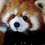# The Laplace transform from scratch

Here's a natural way to derive the Laplace transform via taking the concept of a taylor series and making it continuous. We will, of course, start with a taylor series whose coefficients are given by $a(n)$

$\qquad \qquad \displaystyle\sum_0^{\infty} a(n) x^n \qquad x<1$

Then, make this continuous by replacing the sum by an integral

$\qquad \qquad \displaystyle\int_0^{\infty} a(n) x^n dn \qquad x<1$

We can change $x^n$ to $e^n \ln x$ and make the substitution $s =- \ln x$ to give us

$\qquad \qquad \displaystyle\int_0^{\infty} a(n) e^{-sn} dn \qquad s > 0$

Which is just the definition for the Laplace transform of $a(n)$, $\mathcal{L}(a)(s)$.Note by Levi Walker
1 year, 7 months ago

This discussion board is a place to discuss our Daily Challenges and the math and science related to those challenges. Explanations are more than just a solution — they should explain the steps and thinking strategies that you used to obtain the solution. Comments should further the discussion of math and science.

When posting on Brilliant:

• Use the emojis to react to an explanation, whether you're congratulating a job well done , or just really confused .
• Ask specific questions about the challenge or the steps in somebody's explanation. Well-posed questions can add a lot to the discussion, but posting "I don't understand!" doesn't help anyone.
• Try to contribute something new to the discussion, whether it is an extension, generalization or other idea related to the challenge.

MarkdownAppears as
*italics* or _italics_ italics
**bold** or __bold__ bold
- bulleted- list
• bulleted
• list
1. numbered2. list
1. numbered
2. list
Note: you must add a full line of space before and after lists for them to show up correctly
paragraph 1paragraph 2

paragraph 1

paragraph 2

[example link](https://brilliant.org)example link
> This is a quote
This is a quote
    # I indented these lines
# 4 spaces, and now they show
# up as a code block.

print "hello world"
# I indented these lines
# 4 spaces, and now they show
# up as a code block.

print "hello world"
MathAppears as
Remember to wrap math in $$ ... $$ or $ ... $ to ensure proper formatting.
2 \times 3 $2 \times 3$
2^{34} $2^{34}$
a_{i-1} $a_{i-1}$
\frac{2}{3} $\frac{2}{3}$
\sqrt{2} $\sqrt{2}$
\sum_{i=1}^3 $\sum_{i=1}^3$
\sin \theta $\sin \theta$
\boxed{123} $\boxed{123}$

Sort by:

Nice!

- 1 year, 3 months ago